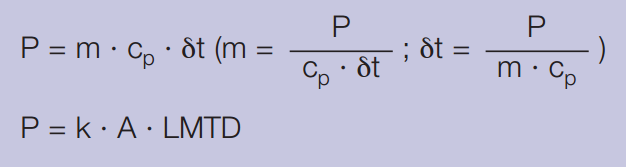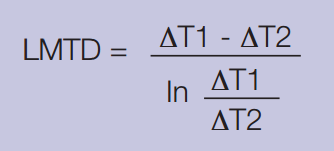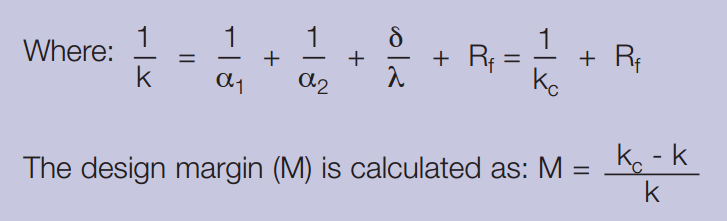#To solve a thermal problem, we need to know several parameters. Further data can then be determined.

The six most important parameters include:

• The amount of heat to be transferred (heat load)
• The inlet and outlet temperatures on the primary and secondary sides
• The maximum allowable pressure drop on the primary and secondary sides
• The maximum operating temperature
• The maximum operating pressure
• The flowrate on the primary and secondary sides

If the flow rate, specific heat and temperature difference on one side are known, the heat load can be calculated.

## How do you calculate the heat transfer area of a plate heat exchanger?

The heat load of a heat exchanger can be derived from the following two formulas:

### 1. Heat load, Theta and LMTD calculation#### Where:

m = mass flow rate (lb/h)

cp = specific heat (btu/lb °F)

δt = temperature difference between inlet and outlet on one side (°F)

k = heat transfer coefficient (btu/ft2 h °F)

A = heat transfer area (ft2)

LMTD = log mean temperature differenceT1 = Inlet temperature - hot side

T2 = Outlet temperature - hot side

T3 = Inlet temperature - cold side

T4 = Outlet temperature - cold side

#### LMTD can be calculated by using the following formula, where ∆T1 = T1–T4 and ∆T2 = T2–T3### 2. Heat transfer coefficient and design margin

The total overall heat transfer coefficient k is defined as:α1 = The heat transfer coefficient between the warm medium and the heat transfer surface (btu/ft2 h °F)

α2 = The heat transfer coefficient between the heat transfer surface and the cold medium (btu/ft2 h °F)

δ = The thickness of the heat transfer surface (ft)

Rf = The fouling factor (ft2 h °F/btu)

λ = The thermal conductivity of the material separating the medias (btu/ft2 h °F)

kc = Clean heat transfer coefficient (Rf=0) (btu/ft2 h °F)

k = Design heat transfer coefficient (btu/ft2 h °F)

M = Design Margin (%)

Combination of these two formulas gives: M = kc · Rf

i.e the higher kc value, the lower Rf-value to achieve the same design margin.

For a more complete explanation of heat transfer theory and calculations, download the following brochure:

The theory behind heat transfer